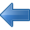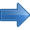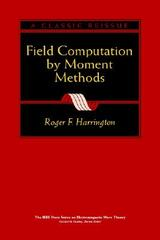Electrostatic Applications Books by Topic Author IndexPrevious Computer and mathematical models Next# Field Computation by Moment Methods

## Roger F. Harrington

1993

240 pages

An IEEE reprinting of this classic 1968 edition, FIELD COMPUTATION BY MOMENT METHODS is the first book to explore the computation of electromagnetic fields by the most popular method for the numerical solution to electromagnetic field problems. It presents a unified approach to moment methods by employing the concepts of linear spaces and functional analysis. Written especially for those who have a minimal amount of experience in electromagnetic theory, this book illustrates theoretical and mathematical concepts to prepare all readers with the skills they need to apply the method of moments to new, engineering-related problems. Written especially for those who have a minimal amount of experience in electromagnetic theory, theoretical and mathematical concepts are illustrated by examples that prepare all readers with the skills they need to apply the method of moments to new, engineering-related problems.

Contents
Deterministic Problems
• Introduction
• Formulation of Problems
• Method of Moments
• Point Matching
• Subsectional Bases
• Approximate Operators
• Extended Operators
• Variational Interpretation
• Perturbation Solutions
Electrostatic Fields
• Operator Formulation
• Charged Conducting Plate
• Conductors of Complex Shape
• Arbitrary Excitation of Conductors
• Electric Polarizability
• Dielectric Bodies
Two-Dimensional Electromagnetic Fields
• Transverse Magnetic Fields
• Conducting Cylinders, TM Case
• Various Approximations
• Transverse Electric Fields
• Conducting Cylinders, TE Case
• Alternative Formulatio
• Dielectric Cylinders
Wire Antennas and Scatterers
• Formulation of the Problem
• Matrix Solution
• Evaluation of Zmn
• Wire Antennas
• Wire Scatterers
• Discussion
Generalized Network Parameter
• Conducting Bodies
• Point-fed Antennas
• Conducting Scatterers
• Aperture Antennas
• Dielectric Bodies
• Magnetic Bodies
• Bodies both Magnetic and Dielectric
Multiport Systems
• Network Representation
• Multiple Feeds and Loads
• Multiply Loaded Scatterers
Eigenvalue Problems
• Introduction
• Method of Moments
• Nonuniform Transmission Lines
• Second-order Differential Operator
• First-order Differential Operator
• Extended Operators
Cylindrical Waveguides
• Second-order Differential Equation
• Second-order Difference Operato
• Moment Solutions
• Extended Operators
• First-order Differential Equations
• Moment Solutions
• Extended Operators
• Use of Generalized Impedances
Cavity Resonators
• Statement of the Problem
• Moment Solution
• Plasma-filled Rectangular Cavity
• Numerical Results
• Discussion
Optimization
• Hermitian Forms
• Optimization Procedure
• Antenna Gain
• Absorption Area
• Bandwidth and Q
• Experimental Gain Optimization

Appendix
• Linear Spaces and Mapping
• Matrix Inversion
• Matrix Eigenvalue and Eigenvectors